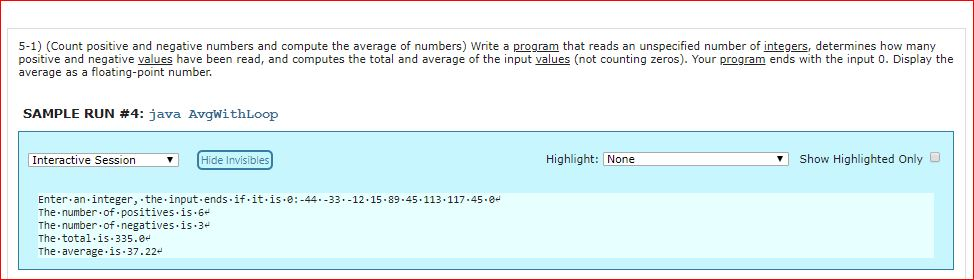# 5-1) (Count positive and negative numbers and compute the average of numbers) Write a program that reads an unspecified number of integers, determines how manypositive and negative values have been read, and computes the total and average of the input values (not counting zeros). Your program ends with the input 0. Display theaverage as a floating-point numberSAMPLE RUN #4: java AvgwithLoopInteractive SessionHide InvisiblesHighlight: NoneShow Highlighted Only DEnter.an.integer, the.input ends.if.it.is.e:-4433.-12.15.89.45The-number-of. positives is . 6The number.of.negatives.is.3The total.is.335.0The average.is 37.22113.117.45.8

Question
847 views

5-1) (Count positive and negative numbers and compute the average of numbers)

Write a program that reads an unspecified number of integers, determines how many positive and negative values have been read, and computes the total and average of the input values (not counting zeros). Your program ends with the input 0. Display the average as a floating-point number.help_outlineImage Transcriptionclose5-1) (Count positive and negative numbers and compute the average of numbers) Write a program that reads an unspecified number of integers, determines how many positive and negative values have been read, and computes the total and average of the input values (not counting zeros). Your program ends with the input 0. Display the average as a floating-point number SAMPLE RUN #4: java AvgwithLoop Interactive SessionHide Invisibles Highlight: None Show Highlighted Only D Enter.an.integer, the.input ends.if.it.is.e:-4433.-12.15.89.45 The-number-of. positives is . 6 The number.of.negatives.is.3 The total.is.335.0 The average.is 37.22 113.117.45.8 fullscreen
check_circle

Step 1

Java program to count posive and negative numbers and computing the total and average of numbers is as follows:

Step 2

Program:

import java.util.*;

//Declare the class name
public class CountNumbers
{
//Main method
publicstaticvoid main(String[] args)
{
//Scanner object for input
Scanner sc = new Scanner (System.in);

//Declare the becessary variables
float tot = 0;
double avg = 0.0;
int neg = 0;
int pos = 0;
int numbers = 0;
int n;

//Print statement
System.out.println("Enter an integer, the input"+"ends if it is 0:");

//Get the input from the user
n = sc.nextInt();

//Read an unspecified number of itegers
while(n!=0)
{
//Check the condition
if (n > 0)

//True, increment it by 1
pos++;

//Check the condition
elseif(n<0)

//True, increment it by 1
neg++;

//Increment the numbers by 1
numbers++;

//Add the value to the total
tot += n;

//Read an unspecified number of itegers
n = sc.nextInt();
}

//Check the condition
if(numbers == 0)
{
//True, print statement
System.out.println("No n...

### Want to see the full answer?

See Solution

#### Want to see this answer and more?

Solutions are written by subject experts who are available 24/7. Questions are typically answered within 1 hour.*

See Solution
*Response times may vary by subject and question.
Tagged in

### Computer Science